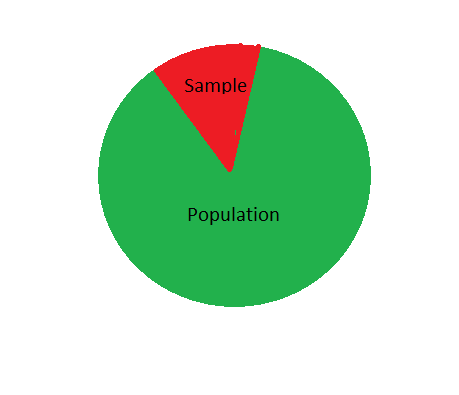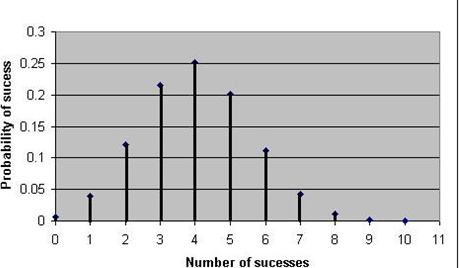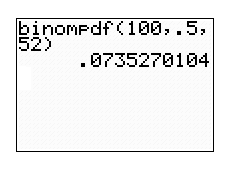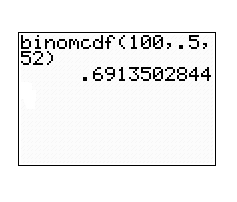# Binomial Random Variables and Binomial Distribution – Probability | Class 12 Maths

• Last Updated : 02 Aug, 2021

A Binomial random variable can be defined by two possible outcomes such as “success” and a “failure”. For instance, consider rolling a fair six-sided die and recording the value of the face. The binomial distribution formula can be put into use to calculate the probability of success for binomial distributions. Often it states “plugin” the numbers to the formula and calculates the requisite values.

The binomial distribution is based upon the following characteristics:

• The experiment contains n identical trials.
• Each trial results in one of the two outcomes either success or failure.
• The probability of success, denoted p, remains the same from trial to trial.
• All the n trials are independent.

### Recognize the Binomial Variable

In order to recognize the binomial variable, the following conditions are applicable:-

• Fixed number of trials.
• Every trial is independent of one another.
• Each trail has two possibilities.
• Success
• Failure
• Probability for success is defined by (p) and probability for failure is defined by (1-q).

For Example, consider the following instance

A fair coin is flipped 20 times; X represents the number of heads.

X is binomial with n = 20 and p = 0.5.

If the above four conditions are satisfied then the random variable (n)=number of successes(p) in trials is a binomial random variable with

• The Mean (Expected Value) is: μ = Σxp
• The Variance is: Var(X) = Σx2p − μ2
• The Standard Deviation is: σ = √Var(X)

### Ten Percent Rule of Assuming Independence

The 10% condition of assuming independence defines that sample sizes should be no more than 10% of the population.The 10% Rule of Assuming Independence condition normally applies to the following cases:-

• While drawing samples without replacement. For Example, In the Central Limit Theorem.
• When proportions from two groups are there
• Solving differences of means for very small populations or an extremely large sample.
• While dealing with Bernoulli trials.

Note: Usually,10% of conditions mentioned won’t find statistical means. For means, the samples are usually smaller, making the condition necessary only if sampling from a very small population.

The condition applies in Bernoulli trials, where the vast majority of cases you sample without replacement.

### Binomial Distribution

A binomial distribution is defined as the probability of a SUCCESS or FAILURE outcome in an experiment that is repeated multiple times. For example, in a coin toss experiment heads or tails and taking a test could have two possible outcomes- Pass or Fail.

In the binomial formula, the number of times the experiment runs is denoted by n

The probability of one specific outcome is denoted by p.

For Example, In an experiment to know the probability of getting a 5 on a die roll. If you were to roll a die 10 times, the probability of rolling a one on any throw is 1/6. Roll ten times and you have a binomial distribution of (n = 10, p = 1/6).

SUCCESS = if the roll is 5 on a die.

FAILURE = if the roll on die is other than 5.

The binomial distribution formula is:

b(x; n, P) = nCx * Px * (1 – P)n – x

where:

• b = defined as a binomial probability
• x = It is the total number of “successes” (pass or fail, heads or tails, etc.)
• P = The probability of success on an individual trial
• n = Defines the number of trials

Note: The above mentioned Binomial Formula can also be written as :

nCx = n! / x!(n – x)!

### Visualization of a Binomial Distribution

Consider a trial of n Independent binomial distribution. SUCCESS in ‘n’ independent is trials is defined by probability ‘p’ in binomial distribution with parameters n and p.

For Instance, in an experiment of tossing a fair coin. The number of heads in 20 tosses of a coin has a binomial distribution with parameters

n = 20 and p = 50%.

The expected value of the binomial distribution is defined as follows:

n × p

The standard error of the binomial distribution is defined as

(n × p × (1 – p))½

If the following four conditions are met then the random variable is binomial:

• Fixed number of trials = n
• Each trial has two possible outcomes:
• success
• failure.
• The probability of success is stated = p
• All the trials should be independent which means that one trail output should not depend on others.

### Binomial Distribution Example

In an experiment of tossing a fair coin 10 times, then the probability of getting exactly 6 heads can be calculated as:

b(x; n, P) – nCx * Px * (1 – P)n – x

Example:

The number of trials (n) = 10

Success (“tossing ahead”) = 0.5

q= 1 – p = 0.5, x = 6

Solution:

P(x = 6) = 10C6 * 0.5^6 * 0.5^4

= 210 * 0.015625 * 0.0625

P(x = 6) = 0.205078125

### Generalizing k scores in n attempts

Binomial Probability Formula :then solving the above results into,It can also simply written as,

P(X = r) = Combinations × P(yes) × P(no)

The combinations, here’s the formula:### Derivation of Binomial Distribution

The outcomes of the binomial distributions are usually depicted as SUCCESS and FAILURE. The usual notations which are used to denote such notations are

• p = probability of success,
• q = probability of failure = 1 – p

Therefore, p + q = 1.

In a Bernoulli trial, each repetition of an experiment involves only 2 outcomes.

1. Independent – In this, the result of one trial does not affect the result of another trial.
2. Repeated – In this, conditions remain the same in each trial. It states that p and q remain constant across trials.

In a binomial distribution,

The probabilities of interest are those of receiving a certain number of successes = r,

In n independent trials each having only two possible outcomes and the same probability, p, of success.

• Following defined is the number of different ways which shows N distinct things may be arranged in order is
N! = (1)(2)(3)...(N-1)(N), (where 0! = 1)
• Below mentioned are the number of ways of selecting r distinct combinations of N objects, irrespective of a valid order, is represented asLet’s apply the formula binom{n-1}{k-1} to this expression and simplify the result is shown below:Furthermore,Following the properties of the factorial function as shown below:Use the above formula to derive the property of the binomial coefficient,now using the commutative property of multiplication (x . y = y . x), the right-hand side can be rewritten as,Now, using the above equations we can equate,Now, the final identity can be concluded as,### Free Throw Binomial Distribution

Consider a scenario to find the best free-throw shooter on your high school basketball team. To find a season free throw percentage, Free Throw Binomial Distribution is put into use

For this, consider an example of Graphing Binomial Distribution:

Consider variable p that will represent the free throw percentage of your best free throw shooter. If the accuracy of the shooting throw is 90%

Then p = 0.9,  p represents the probability of a “success”

To find the probability the athlete will make 3 out of 10 shots if his free throw percentage is 90%.

• Using the binomial formula:
b(x; n, P) – nCx * Px * (1 – P)n – x
• Using the calculator:  binompdf (10, 0.90, 3).

Example:similarly, by calculating values from P(X = 2) to P(X = 10) following values are obtained :

Solution:

Considering the above values following graph is obtained:Binomial distribution n=10, p=2

### Binompdf and binomcdf Functions

The binompdf function on TI 83 or 84 calculators is used for finding the probability of exactly some number of successes.

P(X = c) = binompdf(n, p, c)

where,

n = number of trails

p = number of success

c = probability of exactly c success, for number c.

Example: A fair coin is tossed 100 times. What is the binompdf probability that heads will appear exactly 52 times?

Solution: Using the Binomial formula= binompdf (number of trials, probability of occurrence, number of specific events)

= binompdf (n, p, r)The binomcdf function on the TI-84 calculator. It can be used to solve problems where probability is less than or equal to a number of successes out of a certain number of trials.

Example: To calculate the probability of less than or equal to 45 successes out of 100 trials, the following method is used;

P(X = c) = binomcdf(n, p, c)

where,

n = the number of trials

p = the probability of success for any particular trial

c =  number of successes.

Example 4: A fair coin is tossed 100 times. What is the probability binomcdf there will be at most 52 heads?

Solution:

binomcdf (number of trials, probability of occurrence, number of specific events)

= binomcdf (n, p, r)Example: In a burger shop 70% of people preferred to eat a non-veg burger while others prefer to eat something else. What is the probability of selling 2 non-veg burgers to the next 3 customers?

Solution:

The probabilities for “non-veg burger” all work out to be 0.147 because

0.147 = 0.7 × 0.7 × 0.3

Or, using exponents:= 0.72 × 0.31

The 0.7 is the probability of each choice we want, call it p

The 2 is the number of choices we want, call it k= pk × 0.31

The 0.3 is the probability of the opposite choice, so it is: 1−p

Using formula = pk(1 – p)(n – k)

Where p is the probability of each choice we want

k is the number of choices we want

n is the total number of choices

p = 0.7 (chance of non-veg burger)

k = 2 (non-veg burger choices)

n = 3 (total choices)

So we get: pk(1-p)(n-k) = 0.72(1-0.7)(3-2)

= 0.72(0.3)(1)

= 0.7 × 0.7 × 0.3

= 0.147

Three other possibilities are : (non-veg, non-veg, other) or (non-veg, other, non-veg) or (other, non-veg, non-veg)

n!k! / (n-k)!  = 3!2!(3-2)!

= 3×2×12×1 × 1

= 3

3  (Number of outcomes we want ) ×   0.147  (Probability of each outcome    ) =   0.441

So, 70% choose non-veg burger, so 7 of the next 10 customers should choose non-veg burger

p = 0.7

n = 10

k = 7

And we get:  pk(1-p)(n-k) = 0.77(1-0.7)(10-7)

= 0.77(0.3)(3)

= 0.0022235661

And the total number of those outcomes is:

n!k!/(n-k)! =10!7!/(10-7)!

= 10×9×8×7×6×5×4×3×2×17×6×5×4×3×2×1 × 3×2×1

= 10×9×83×2×1

=120

120 (Number of outcomes we want) × 0.0022235661(Probability of each outcome ) = 0.266827932

So the probability of 7 out of 10 choosing a non-veg burger is only about 27%

My Personal Notes arrow_drop_up